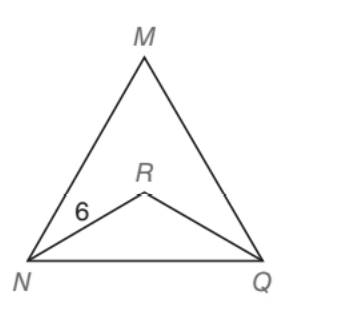Chapter 5.5, Problem 29EElementary Geometry For College St...

7th Edition
Alexander + 2 others
ISBN: 9781337614085

Solutions

Chapter
SectionElementary Geometry For College St...

7th Edition
Alexander + 2 others
ISBN: 9781337614085
Textbook ProblemTo determine

To find:

NQ, in the MNQ, which is an equianglular with NR=6 and NR bisects MNQ, QR bisects. MQN

Explanation

Approach:

For a right triangle, for which the measure of the interior angles 30°, 60°, and 90°; if ‘a’ is the length of measure of the shorter leg; opposite to the angle 30°, then the length of the other two sides is given by

Length of the longer leg (opposite to 60°) =a3

Length of the hypotenuse (opposite to 90°)=2a.

In general

Length of the longer leg =3× (Length of the shorter leg)

Length of the hypotenuse =2× (Length of the shorter leg)

Calculation:

Given,

A equiangular triangle MNQ with NR bisects MNQ, QR bisects MNQ.

NR=6.

MNQ, is an equiangular triangle.

Thus,

mM=60°

mN=60°, and

mQ=60°

Now, the angle MNQ and MQN are bisected by the rays NR and QR respectively.

mRNQ=12·mMNQ

=12(60°)

mRNQ=30°

Also,

c12·mMQN

=12(60°)

mRQN=30°

Now, consider the right triangle RNQ of the above diagram.

We have

mN=mQ=30°

Thus, by the theorem that the measure of the sides opposite to the congruent angles are congruent, we have

NR¯RQ¯

Thus,

NR=RQ=6 units

Still sussing out bartleby?

Check out a sample textbook solution.

See a sample solution

The Solution to Your Study Problems

Bartleby provides explanations to thousands of textbook problems written by our experts, many with advanced degrees!

Get Started

Find the mean, median, and mode for the following scores; 8, 7, 5, 7, 0, 10, 2, 4, 11, 7, 8, 7

Essentials of Statistics for The Behavioral Sciences (MindTap Course List)

Find the maximum profit P (in dollars) given that 6(P 2500) 4(P + 2400)

Applied Calculus for the Managerial, Life, and Social Sciences: A Brief Approach

Write the following numbers in word form. .61

Contemporary Mathematics for Business & Consumers

True or False: The Alternating Series Test may be applied to determine the convergence of n=12+(1)n2n2.

Study Guide for Stewart's Single Variable Calculus: Early Transcendentals, 8th